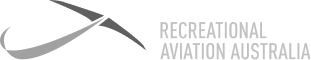# Definition: Density Altitude (DA)

This is the amount of air in a given space above mean sea level (AMSL). In other words, the more dense the air is, the more the amount of air in a given amount of space. Density altitude is pressure altitude corrected for temperature. As density altitude increases, aircraft performance decreases.### Density Altitude (DA)

This is the amount of air in a given space above mean sea level (AMSL). In other words, the more dense the air is, the more the amount of air in a given amount of space. Density altitude is pressure altitude corrected for temperature. As density altitude increases, aircraft performance decreases.

7 Day

FREE

Trial